# Is right triangle or not

If right triangle ABC, have sides a=13, b=11.5, c=22.5. Find area.

Correct result:

A =  54.415

#### Solution:

$a=13 \ \\ b=11.5 \ \\ c=22.5 \ \\ \ \\ c_{1}=\sqrt{ a^2+b^2 }=\sqrt{ 13^2+11.5^2 } \doteq 17.3566 \ \\ c_{1}<>c \ \\ \ \\ s=\dfrac{ a+b+c }{ 2 }=\dfrac{ 13+11.5+22.5 }{ 2 }=\dfrac{ 47 }{ 2 }=23.5 \ \\ \ \\ A=\sqrt{ s \cdot \ (s-a) \cdot \ (s-b) \cdot \ (s-c) }=\sqrt{ 23.5 \cdot \ (23.5-13) \cdot \ (23.5-11.5) \cdot \ (23.5-22.5) }=3 \ \sqrt{ 329 }=54.415$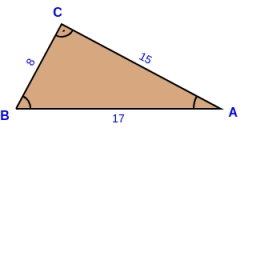Our examples were largely sent or created by pupils and students themselves. Therefore, we would be pleased if you could send us any errors you found, spelling mistakes, or rephasing the example. Thank you!

Leave us a comment of this math problem and its solution (i.e. if it is still somewhat unclear...):Be the first to comment!Tips to related online calculators
Pythagorean theorem is the base for the right triangle calculator.

#### You need to know the following knowledge to solve this word math problem:

We encourage you to watch this tutorial video on this math problem:

## Next similar math problems:

• Parallelogram perimeter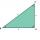The ABC triangle with sides a = 5cm, b = 3cm, c = 40mm has the center of the sides of the K,L,M. How many cm have the KBLM parallelogram perimeter?
• Chauncey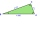Chauncey is building a storage bench for his son’s playroom. The storage bench will fit into the corner and against two walls to form a triangle. Chanuncy wants to buy a triangular shaped cover for the bench. If the storage bench is 2 1/2 ft. Along one
• DiagonalsDraw a square ABCD whose diagonals have a length of 6 cm
• Surface area 6Find the surface area of a prism whose bases are right triangles with sides of length 3, 4, and 5 inches and a height of 8 inches. Include a sketch
• MidpointsTriangle ABC with sides a = 5 cm, b = 3 cm, c = 40mm has a midpoint of K, L, M. How many centimeters is long perimeter of parallelogram KBLM?
• Surface area of the topA cylinder is three times as high as it is wide. The length of the cylinder’s diagonal is 20 cm. Find the surface area of the top of the cylinder.
• Complementary angles 2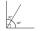Two complementary angles are (x+4) and (2x - 7) find the value of x
• Triangular prismCalculate a triangular prism if it has a rectangular triangle base with a = 4cm and hypotenuse c = 50mm and height of the prism is 0.12 dm.
• Cube wallCalculate the cube's diagonal diagonal if you know that the surface of one wall is equal to 36 centimeters square. Please also calculate its volume.
• SquareCalculate the perimeter and the area of square with a diagonal length 30 cm.
• Horizontal distance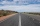The road has a gradient of 8%. How many meters will the road rise on a horizontal distance of 400m?
• Perpendicular prism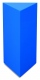Calculate the volume of the perpendicular prism if its height is 60.8 cm and the base is a rectangular triangle with 40.4 cm and 43 cm legs.Miro stands under a tree and watching its shadow and shadow of the tree. Miro is 180 cm tall and its shade is 1.5 m long. The shadow of the tree is three times as long as Miro's shadow. How tall is the tree in meters?A 2-meter rod casts a shadow 3.2 m long. How high is a tree with a shadow of 14.4 m ?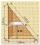Place a glass shelf at the height of 1m from the bottom of the display case in the cabinet. How long platter will we place at this height? The display case is a rectangular triangle with 2 m and 2.5 m legs.A 68 centimetre long rope is used to make a rhombus on the ground. The distance between a pair of opposite side corners is 16 centimetres what is the distance between the other two corners?Ladder 10 meters long is staying against the wall so that its bottom edge is 6 meters away from the wall. What height reaches ladder?